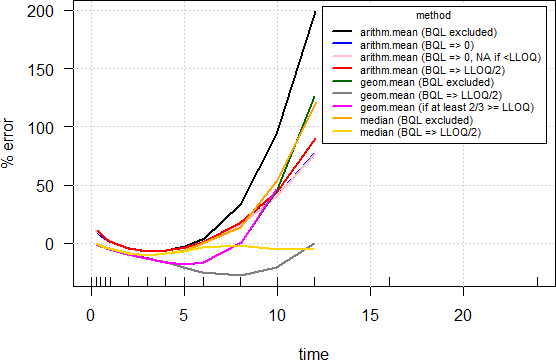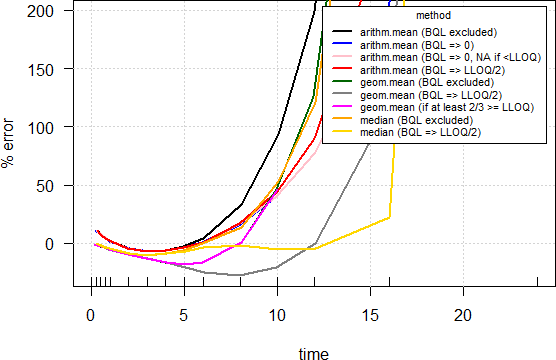## “Average” plots [NCA / SHAM]

Dear all,

following this thread and esp. this post I hijacked Mittyri’s simulation code another time. As nobody rightly wrote

❝ Statistics is always some kind of abstraction.

Why are we using average* plots at all? The complete information is contained in spaghetti plots but Ohlbe remarked

❝ I find spaghetti plots rather difficult to read with more than 30 subjects, or even less depending on what they look like…

So which kind of average plot would allow a significant data reduction (one line per treatment) instead of one for every subject? Ideally it should convey unbiased information. I simulated 1,000 subjects and calculated averages as described there. It set the “LLOQ” of the method to 2% of the theoretical Cmax. If a concentration was calculated as BQL, I set it to NA. At the end I compared the averages with the theoretical concentrations at all time points i, i.e., calculated $$\small{\%RE=100\big{(}(\bar{x}_i-C_i)/C_i\big{)}}$$. Plotting $$\small{\%RE}$$ vs. $$\small{t}$$ should give an idea which one to choose. I tried two flavors:
1. If the theoretical concentration C would be <LLOQ I forced it to NA.
2. I used it ‘as is’, which means that at 16 and 24 hours it is still used though it is far below the LLOQ.
Outcome:
1. All methods based on the arithmetic mean are terrible. Nothing new. The median where BQLs are imputed with LLOQ/2 seems to be the winner (always a moderate negative bias). Since the theoretical concentrations are BQL after 12 hours, no comparison possible.2. Pretty similar but all go through the ceiling. Useless but gives an idea that we should take average curves in the terminal phase approaching the LLOQ with a grain of salt.• Average as a general term for a measure of location (mean, median, …).-script
C <- function(F=1, D, Vd, ka, ke, t) {   C <- F*D/Vd*(ka/(ka - ke))*(exp(-ke*t) - exp(-ka*t))   return(C) } loc.stat <- function(x, type, na.rm) {   non.numerics    <- which(is.na(suppressWarnings(as.numeric(x))))   x[non.numerics] <- NA   x <- as.numeric(x)   switch(type,          arith.mean = round(mean(x, na.rm=na.rm), 2),          median     = round(median(x, na.rm=na.rm), 2),          geom.mean  = round(exp(mean(log(x), na.rm=na.rm)), 2)) } Nsub     <- 1000 D        <- 400 ka       <- 1.39 ka.omega <- 0.1 Vd       <- 1 Vd.omega <- 0.2 CL       <- 0.347 CL.omega <- 0.15 t        <- c(seq(0, 1, 0.25), seq(2,6,1), 8,10,12,16,24) ke       <- CL/Vd C.th     <- C(D=D, Vd=Vd, ka=ka, ke=ke, t=t) tmax     <- log((ka/ke)/(ka - ke)) Cmax     <- C(D=D, Vd=Vd, ka=ka, ke=ke, t=tmax) LLOQ.pct <- 2 # LLOQ = 2% of theoretical Cmax LLOQ     <- Cmax*LLOQ.pct/100 df1      <- data.frame(t=t) for (j in 1:Nsub) {   ka.sub <- ka * exp(rnorm(1, sd = sqrt(ka.omega)))   Vd.sub <- Vd * exp(rnorm(1, sd = sqrt(Vd.omega)))   CL.sub <- CL * exp(rnorm(1, sd = sqrt(CL.omega)))   df1    <- cbind(df1, C(D=D, Vd=Vd.sub, ka=ka.sub, ke=CL.sub/Vd.sub, t=t))   df1[which(df1[, j+1] < LLOQ), j+1] <- NA } names(df1)[2:(Nsub+1)] <- paste0("S.", 1:Nsub) df2 <- data.frame(t(df1[-1])) colnames(df2) <- df1[, 1] names(df2) <- t loc <- data.frame(method=c("arithm.mean (BQL excluded)",                            "arithm.mean (BQL => 0)",                            "arithm.mean (BQL => 0, NA if <LLOQ)",                            "arithm.mean (BQL => LLOQ/2)",                            "geom.mean (BQL excluded)",                            "geom.mean (BQL => LLOQ/2)",                            "geom.mean (if at least 2/3 >= LLOQ)",                            "median (BQL excluded)",                            "median (BQL => LLOQ/2)"),                   t.1=NA, t.2=NA, t.3=NA, t.4=NA, t.5=NA, t.6=NA, t.7=NA,                   t.8=NA, t.9=NA, t.10=NA, t.11=NA, t.12=NA, t.13=NA, t.14=NA,                   t.15=NA, stringsAsFactors=FALSE) names(loc)[2:16] <- t for (j in 2:(ncol(df2)+1)) {    x <- df2[, j-1]    loc[1, j] <- loc.stat(x, "arith.mean", TRUE)    y <- x    y[which(is.na(x))] <- 0    loc[2, j] <- loc.stat(y, "arith.mean", FALSE)    ifelse(loc[2, j] >= LLOQ, loc[3, j] <- loc[2, j], loc[3, j] <- NA)    y <- x    y[which(is.na(x))] <- LLOQ/2    loc[4, j] <- loc.stat(y, "arith.mean", FALSE)    loc[5, j] <- loc.stat(x, "geom.mean", TRUE)    loc[6, j] <- loc.stat(y, "geom.mean", FALSE)    if (length(which(!is.na(x))) >= nrow(df2)*2/3) {       loc[7, j] <- loc[5, j]    } else {       loc[7, j] <- NA    }    loc[8, j] <- loc.stat(x, "median", TRUE)    loc[9, j] <- loc.stat(y, "median", FALSE) } err2 <- err1 <- loc C <- C.th C[C.th < LLOQ] <- NA for (j in 1:nrow(loc)) {    err1[j, 2:ncol(err1)] <- signif(100*(loc[j, 2:ncol(loc)]-C)/C, 4) } C <- C.th for (j in 1:nrow(loc)) {    err2[j, 2:ncol(err2)] <- signif(100*(loc[j, 2:ncol(loc)]-C)/C, 4) } print(err1, row.names=FALSE);print(err2, row.names=FALSE) clr <- c("black", "blue", "pink", "red", "darkgreen",          "gray50", "magenta", "orange", "gold") dev.new(record=TRUE) plot(x=t, y=rep(0, length(t)), type="n", xlim=range(t),      ylim=range(err1[, 2:16], na.rm=TRUE), xlab="time",      ylab="% error", las=1) grid(); rug(t) for (j in 1:nrow(err1)) {   lines(jitter(t, factor=1.5), err1[j, 2:ncol(err1)], col=clr[j], lwd=2) } legend("topright", bg="white", inset=0.02, title="method",        legend=loc[, 1], lwd=2, col=clr, cex=0.65) plot(x=t, y=rep(0, length(t)), type="n", xlim=range(t),      ylim=range(err1[, 2:16], na.rm=TRUE), xlab="time",      ylab="% error", las=1) grid(); rug(t) for (j in 1:nrow(err2)) {   lines(jitter(t, factor=1.5), err2[j, 2:ncol(err2)], col=clr[j], lwd=2) } legend("topright", bg="white", inset=0.02, title="method",        legend=loc[, 1], lwd=2, col=clr, cex=0.65)

Dif-tor heh smusma 🖖🏼 Довге життя Україна!Helmut SchützThe quality of responses received is directly proportional to the quality of the question asked. 🚮
Science QuotesIng. Helmut Schütz